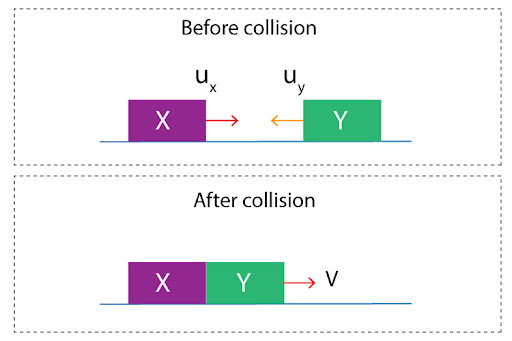# "Two trains are heading in opposite directions on the same track. Train X- mass = 16000kg & speed = 2.8m/s. Train Y- mass = 12000kg & speed = 3.1m/s. At what speed do the joined trains move off together immediately after the collision?"

In this scenario, the two trains approach each other, and then after collision “stick” together. This is an example of inelastic collisions, wherein kinetic energy is not conserved, hence why the two trains move off with the same velocity:To solve this, we will use the principle of conservation of momentum, that the total momentum before and after collision will remain the same:

PT(before)=PT(after)

Before collision:
PT(before)=mxux+myuy

=(16000 2.8) + (12000 (-3.1))

=7600 Ns

After collision:
PT(after) =(mx+my)v

= (16000+12000)v
Equating the two, we solve:
7600 = 28000v

v = 7600/28000=0.271 m/s